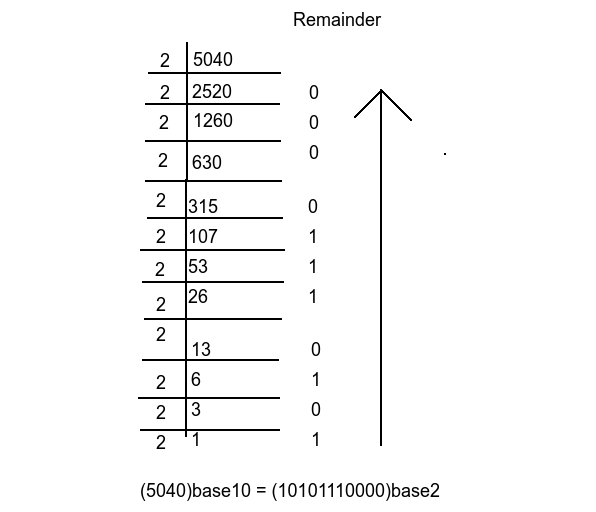# Find the Number of Trailing Zeroes in base B Representation of N! using C++

C++Server Side ProgrammingProgramming

#### C in Depth: The Complete C Programming Guide for Beginners

45 Lectures 4.5 hours

#### Practical C++: Learn C++ Basics Step by Step

Most Popular

50 Lectures 4.5 hours

#### Master C and Embedded C Programming- Learn as you go

66 Lectures 5.5 hours

In this article, we will understand the problem of finding trailing zeros of a given number N in the base B representation of its factorial. For examples

Input : N = 7 Base = 2
Output : 4
Explanation : fact(7) = 5040 in base10 and 1001110110000 in base16 having 4 trailing zero.

Input : N = 11 Base = 5
Output : 2
Explanation : fact(11) = 39916800 in base10 and 40204314200 in base16 having 2 trailing zeroes.

Let's first recap the process of converting any decimal number from one base to another. Let's take an example of converting (5040)10 to (?)2i.e., dividing the number by 2 and keeping the remainder until the number cannot be further divided. The result will be the remainder in reverse order.

As a result, we have 4 trailing zero, and this trailing zero we got when 2 divides the number with remainder 0.

Prime factorization of 5040 = 24 * 56711 * 3381 * 181 that means 2 divides 5040 4 times with remainder 0 which is equal to trailing zeroes. In this way, we can calculate the number of trailing zeroes.

## Approach to find The Solution

We discussed above the way to find the number of trailing zeroes. We need to find the highest power of B in factorial N, let's say base B = 14, then 14 in base 14 will be represented as 10, i.e. (14)10 = (10)14. It is also known as Legendre's formula.

## C++ Code for the Above Approach

Here is the C++ syntax which we can use as an input to solve the given problem −

## Example

#include <bits/stdc++.h>
using namespace std;

vector < pair < int, int >> primeFactorsofBase(int Base) {
// declaring factors to store prime factors
// along with occurence in factorisation of Base .
vector < pair < int, int >>factors;

for (int i = 2; Base != 1; i++) {
if (Base % i == 0) {
int count = 0;
while (Base % i == 0){
Base = Base / i;
count++;
}

factors.push_back (make_pair (i, count));
}
}
return factors;
}

int main () {
int N = 11, Base = 5;
// finding the largest power of Base that divides factorial N.
vector < pair < int, int >>prime_factors;
// finding prime factors by primeFactorsofBase() function.
prime_factors = primeFactorsofBase(Base);

int result = INT_MAX;
for (int i = 0; i < prime_factors.size (); i++) {
// calculating minimum power.
int count = 0;
int r = prime_factors[i].first;
while (r <= N){
count += (N / r);
r = r * prime_factors[i].first;
}
result = min (result, count / prime_factors[i].second);
}
//printing trailing zeroes stored in result.
cout << "Number of trailing zeroes: " <<result;
return 0;
}

## Output

Number of trailing zeroes: 2

## Explanation of the above code

• find the largest power of Base using a vector.
• To calculate the largest power, calculate prime factors using vectors to store all prime factors.
• Then calculate the minimum power of all prime factors of Base.
• Finally, printing the result.

## Conclusion

In this article, we solve the problem of finding the number of trailing zeroes in the base B representation of factorial N, which we solve using Legendre’s formula. We also write C++ code to solve the same problem. You can write this code in any other language like Java, C, python, etc. We hope you find this article helpful.